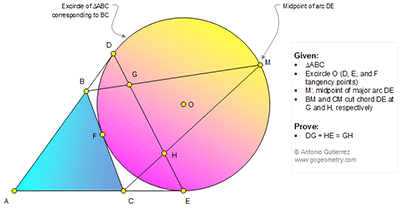## Tuesday, February 21, 2017

### Geometry Problem 1317: Triangle, Excircle, Chord, Tangent, Midpoint, Arc, Sum of two Segments, Congruence

Geometry Problem. Post your solution in the comment box below.
Level: Mathematics Education, High School, Honors Geometry, College.

Details: Click on the figure below.1.Draw C`B` tg to O at F` and // to DE. Join F to H and extend at A` (AE extended)
Draw A`A´` (A`` at AD extended) perpendicular to AO extended, join C` to midpoint of A`A``
Like P1315 => result

2.Join F to H need to be Join F` to H

3.To c.t.e.o

See below for the sketch per your solution as above.

https://goo.gl/photos/ugcUvNraGe6JuUgH7

Suppose that the result of Pr1315 is correct. I don't see how the result of Pr1315 help to prove that DG+HE= GH . please explain

Peter Tran

4.If you complete drawings, join C`, B` to M and C`, B` to midpoint of A`A``
Triangles formed by B`C` and points M and midpoint of A`A`` are isoceles so
segment parts on DE are equal